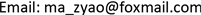1. 引言

2. 结构方程模型理论

{ X 1 = λ 11 ξ 1 + δ 1 X 2 = λ 21 ξ 1 + δ 2 X 3 = λ 31 ξ 1 + δ 3 X 4 = λ 42 ξ 2 + δ 4 X 5 = λ 53 ξ 3 + δ 5 X 6 = λ 63 ξ 3 + δ 6 X 7 = λ 74 ξ 4 + δ 7 X 8 = λ 84 ξ 4 + δ 8 X 9 = λ 94 ξ 4 + δ 9 (1)

{ Y 1 = λ 11 η 1 + ε 1 Y 2 = λ 21 η 1 + ε 2 Y 3 = λ 32 η 2 + ε 3 Y 4 = λ 42 η 2 + ε 4 Y 5 = λ 53 η 3 + ε 5 Y 6 = λ 63 η 3 + ε 6 (2)

{ η 1 = γ 11 ξ 1 + γ 12 ξ 2 + γ 13 ξ 3 + γ 14 ξ 4 + ζ η 2 = γ 21 ξ 1 + γ 22 ξ 2 + γ 23 ξ 3 + γ 24 ξ 4 + β 21 η 1 + ζ 2 η 3 = β 31 η 1 + β 32 η 2 + ζ 3 (3)

3. 模型构建

4. 模型求解

Summary of indicator weights at all level

<---业务办理0.0180.0210.0390.5460.585
<---抄表收费服务0.3010.1890.0218.883***
<---供电安全0.4490.3060.02512.432***
<---电压质量0.1210.1310.0284.688***
<---停电处理0.8250.5240.02619.774***

Data fit summary tabl
ModelPCMIN/DFCFIRMSEAAIC
Default model024.0430.8090.1045046.846

Summary of indicator weights at all level
ModelPCMIN/DFCFIRMSEAAIC
Default model01.9270.9940.021537.813

CMIN/DF < 2，CF I> 0.9，且与1相当接近，RMSEA < 0.05说明模型非常好。

5. 模型分析

Second and third-level indicators mean calculation results summary tabl

95598热线85.09

6. 结论